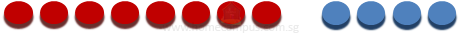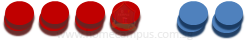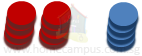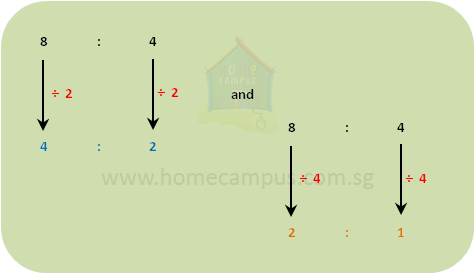## What are Equivalent Ratios?

Practice Unlimited Questions

#### What are Equivalent Ratios?

Ratios that are equal to each other are called equivalent ratios.

Examples:
1.    16 : 4 = 8 : 2 = 4 : 1
2.    1 : 3 = 3 : 6
3.    4 : 25 = 16 : 100 = 160 : 1000
The video tutorial above explains the concept in detail.

#### 1.  Are the ratios 8 : 4, 4 : 2 and 2 : 1 equivalent?

Let's suppose that we have 8 red coins and 4 blue coins.The ratio of the number of red coins to the number of blue coins is 8: 4.

Now, let's rearrange the coins into stacks of 2 coins each as shown below.Now, the ratio of the number of red coins to the number of blue coins is 4 : 2.

Finally, let's put the coins into stacks of 2 coins each as shown below.The new ratio of the number of red coins to the number of blue coins is 2 : 1.For every 8 red coins there are 4 blue coins.

Or

For every 4 red coins there are 2 blue coins.

Or

For every 2 red coins there is 1 blue coin.

The ratios 8 : 4, 4 :2 and 2 : 1 are, therefore, equivalent ratios.

2:1 is the simplest form of 8:4.

Note:
To get the equivalent of a ratio, multiply / divide all the terms of the ratio by the same number.